Reach Us+44-7482-875032
Reliability Analysis for Monte Carlo Simulation Using the Expectation- Maximization Algorithm for a Weibull Mixture Distribution Model | OMICS International
Journal of Applied & Computational Mathematics
All submissions of the EM system will be redirected to Online Manuscript Submission System. Authors are requested to submit articles directly to Online Manuscript Submission System of respective journal.

# Reliability Analysis for Monte Carlo Simulation Using the Expectation- Maximization Algorithm for a Weibull Mixture Distribution Model

Emad E. Elmahdy*

Department of Mathematics, Science College, King Saud University, Riyadh 11451, P.O. 2455, Saudi Arabia

*Corresponding Author:
Emad E. Elmahdy
Department of Mathematics, Science College
King Saud University, Riyadh 11451, P.O. 2455, Saudi Arabia
Tel: 00966508683753
E-mail: [email protected]

Received date: May 20, 2016; Accepted date: May 20, 2016; Published date: June 27, 2016

Citation: Emad E. Elmahdy (2016) Reliability Analysis for Monte Carlo Simulation Using the Expectation-Maximization Algorithm for a Weibull Mixture Distribution Model. J Appl Computat Math 5:310. doi:10.4172/2168-9679.1000310

Copyright: © 2016 Emad E. Elmahdy. This is an open-access article distributed under the terms of the Creative Commons Attribution License, which permits unrestricted use, distribution, and reproduction in any medium, provided the original author and source are credited.

Visit for more related articles at Journal of Applied & Computational Mathematics

#### Abstract

This paper presents a simulation study of a finite Weibull mixture distribution (WMD) for modelling life data related to system components with different failure modes. The main aim of this study is to compare two analytical methods for estimating the parameters of WMD models, the maximum likelihood estimation (MLE) method using the expectation-maximization (EM) algorithm, [A1] and the non-linear median rank regression (NLMRR) method with the Levenberg-Marquardt algorithm. To perform this comparison, the Monte Carlo simulation technique is implemented to generate several replicates for complete failure data and censored data based on samples of different sizes that follow a two-component WMD. This study showed that MLE using the EM algorithm yields more accurate parameter estimates than the NLMRR method for small or moderate complete failure data samples. This method also converges faster than the NLMRR method for large samples that include censored data.

#### Keywords

Expectation-maximization algorithm; Life data analysis; Maximum likelihood estimation method; Monte Carlo simulation method; Non-linear median rank regression method; Root mean squared error; Weibull mixture distribution; Weibull probability paper

#### Introduction

Survival/reliability analysis plays an important role in medicine, epidemiology, engineering, biology, sociology, economics, and many other fields. The outcome of survival studies is the time to the occurrence of a certain event, which is referred to as the survival time, failure time, lifetime data, or event time, e.g., the recurrence of an illness, death, failure of equipment, births, and divorces. In medical studies, the response variable (survival time) is measured in patients from a specific initial point, e.g., the date of diagnosis, type of treatment, or date of a transplant procedure, until the occurrence of a well-known event, such as the cessation of symptoms, deterioration in the condition of the patient, or death. During life testing analysis in engineering studies, mechanical or electronic components are often placed in an operating state and subjected to life tests in a laboratory setting, where they are observed until each fails and the time to failure is recorded for each component [1,2]. Life data analysis can be used for modelling and interpreting life data related to crowd disasters, terrorism, crime, war, disease spread, and patterns in the evolutionary dynamics of populations to obtain a good overall picture of the actual system’s behaviour [3,4].

In reliability analysis, life testing must be performed to study the failure-time distribution of newly designed equipment. A sample of lifetime data must be taken, where each component operates under certain conditions during a specified time interval until it fails, or not, but this type of testing is very expensive for manufacturers. There are two types of lifetime data: complete data and censored data. Complete data comprise the simplest type of life data where the failure time of each component in the sample is known. These data are obtained by recording the exact time when each component fails. Censoring occurs when a component included in life testing fails during observations but the exact time of failure is unknown. The time intervals for these failures are called interval censored data. In addition, censoring occurs when some components are still operating after a life testing experiment has been terminated. The observed operating times of these components are called right censored data or suspensions . An important task in survival analysis is selecting the best model to represent the distribution of the failure time variable and to determine the dependencies of the failure time variable on other independent variables, such as gender, age, weight, temperature, pressure, or diabetes.

The principal tasks in reliability modelling analysis are: selecting the model, parameter estimation, and model validation. The Weibull distribution model was proposed by Waloddi Weibull  and it has many applications in various fields, such as industry and medicine. Many models have been derived from the two-parameter Weibull distribution, which are called Weibull models . The Weibull family of distributions comprises the most widely used statistical models for lifetime data in survival analysis. Weibull models are applied to many human diseases such as Hodgkin’s disease and cancer data . Different Weibull models exhibit a wide variety of shapes and thus they can represent various characteristics for reliability functions. Finite mixture distributions have numerous applications, which range from the length distributions of fish to the DNA content in the nuclei of liver cells . They are also employed in reliability analysis for modelling heterogeneous lifetime data, which means that it is important to study the mathematical properties of Weibull mixture distributions (WMDs). Weibull models are well known in reliability modelling. Three-parameter Weibull, Weibull mixture, and competing risk models are used frequently for modelling life data. Modelling life data using Weibull models involves five main steps: collecting a sample of life data, plotting the data and interpreting the plot, preliminary model selection, parameter estimation, and goodness of fit tests to select the candidate model. Most of the approaches that apply Weibull modelling to reliability testing for life data initially rely on graphing the cumulative distribution function (CDF) on Weibull plotting paper (WPP), where the CDF for complete or censored lifetime data is calculated using a ranking method, such as the median rank method, Kaplan-Meier, or Benard’s median rank [6,10]. The resulting graph may have different shapes, i.e., a straight line represents the twoparameter Weibull model (standard Weibull model), a convex shape indicates the competing risk model or classic Bi-Weibull, an S-shape that approaches a straight line as the data points become smaller, a concave shape with a cusp and a steep slope followed by a shallow slope indicates the existence of two sub-population distributions (simple mixture distribution) [10,11], and a concave shape with a vertical asymptote and a right asymptote or a line that curves downward at the lower end represents the three-parameter Weibull model. Moreover, different Weibull mixture models of two and three failure modes can include batch effects, as described in [6,7]. Several methods can be employed to obtain parameter estimates for different Weibull models, such as graphics, moments, maximum likelihood estimation (MLE), Bayes estimators, Monte Carlo simulation methods, and MLE using the expectation-maximization (EM) algorithm [12-16]. After estimating the parameters for the selected Weibull models for specific sample data, it is necessary to examine the goodness-of-fit for each. Statistical measures for goodness-of-fit, such as confidence prediction bounds and r-squared denoted by r2 and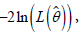where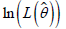is the natural logarithm of the MLE function, are employed to determine the best Weibull model for modelling life data .

When a scientist studies a specific phenomenon, it is necessary to repeat an experiment several times to obtain a sample of measurements or observations of this phenomenon. The aim is to make a general statement about the phenomenon. It is possible that the observations obtained in an experiment may follow a specific pattern, which is known as the probability distribution of a population in probability theory. Monte Carlo simulation is an important technique for generating a random variable that follows a specific statistical probability distribution. Monte Carlo simulation differs from ordinary analytical methods. In analytical methods, the life process of a component or a system is described by a mathematical model such as exponential, Weibull, gamma, or log-normal. The required reliability indices are then estimated. In the Monte Carlo approach, the actual process is simulated on a computer and the desired reliability indices are estimated after observing the simulated process for some time. Thus, the simulation is considered to be a series of real experiments where the events occurring at specific times are determined by random processes, which follow appropriate probability distributions. An important problem with the Monte Carlo simulation method is that various events are constrained in that simulation so they must conform with certain distributions. The simplest way to achieve this for a given event is by selecting a random number from a large set of numbers that belong to the appropriate distribution and making the event “occur” at the moment indicated by the number selected . This method requires the generation and storage of several sets of numbers with distributions that correspond to all of the time distributions involved in the process, but the process can be simplified using a single set where the numbers are distributed uniformly between 0 and 1. A number selected randomly from this set can then be simply converted into a number from a set with an arbitrary distribution using the appropriate CDF. Basically, the Monte Carlo method is a probabilistic method, which is included in many computer software libraries such as Weibull++ and SuperSMITH Weibull (SSW) to generate samples from different Weibull models [6,18]. Thus, a large number of reliability analysis can be performed using the generated data sets and this process may be repeated many times. The Monte Carlo simulation method is very important for exploring the design of reliability tests because it allows us to optimize a model with respect to its parameters, thereby defining many reliability indices, such as a CDF, reliability function R(t), probability density function, mean time to failure of a component for non-repairable systems, or hazard function (failure rate) h(t). The Monte Carlo simulation method helps us to study the influence of sample size, which may include censored data in reliability analysis methods. Thus, many experiments can be executed using life data samples with different sizes for complete and censored data.

In this study, the effects on analysis methods for estimating mixing parameters of generated WMD lifetime data samples with different sizes are examined. Two methods are investigated, i.e., MLE using the EM algorithm and non-linear median rank regression (NLMRR) with the Levenberg-Marquardt algorithm, where the objective function must be of the least squares type with no constraints. The objective of this study is to compare the performance of MLE using the EM algorithm and the NLMRR method. The remainder of this paper is organized as follows. A critical review of WMDs are provided and two methods for estimating the parameters of WMD are introduced: MLE using the EM algorithm and NLMRR. Monte Carlo simulation is employed to compare the performance of the two proposed estimation methods. Monte Carlo simulation is used to generate two types of data: complete failure data and failure data with heavily right censored data for samples of different sizes that follow the two-component WMD. the results are summarized and the conclusions of this study are given.

#### Estimating the Parameters of a WMD Model

Several methods can be applied to estimate the parameters for WMD models. The graphical method can be used for modelling complete and censored life data. For a WMD model, the graph of the data points on WPP is as a concave upward curve with a cusp  or it is S-shaped, which indicates the existence of batch problem (mixture of failure modes). Elmahdy et al. [10,11] proposed an algorithm to estimate the parameters for WMD with complete/censored life data by using MLE with the EM algorithm. This iterative algorithm can be summarized in two steps: the E step estimates the joint likelihood of the observed failure times or censored life data set that follow WMD; and in the M step, the expectation is maximized over the unknown parameter values, where the resulting values for the estimated parameters are used in the next E step. This process is repeated many times until convergence is obtained with sufficient accuracy. Elmahdy et al.  also introduced a new approach for modelling actual life data using different Weibull models, such as three-parameter Weibull, Weibull mixture, and Weibull competing risk models. This approach is efficient for grouped and ungrouped samples of different sizes that include a heavily censored life data set and few exact failure times.

Finite Weibull mixture model

Finite Weibull mixture models are univariate models. The finite Weibull mixture model describes the density f(t|θ) as a combination of m weighted densities, which can be written as follows.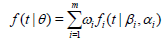(1)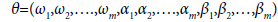is the parameter vector of an m-mixed Weibull distribution where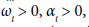and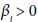denote the mixing weight, scale, and shape parameter of sub-population i respectively,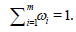The probability density function of the standard Weibull model (two parameter Weibull distribution) for subpopulation i is given by: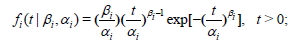(2)

therefore,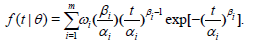(3)

In reliability analysis, the survivor (reliability) function R(t|θ) and the hazard (failure rate) function h(t|θ) of a WMD can be defined as follows.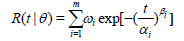(4)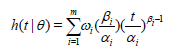(5)

MLE using the EM Algorithm for estimating WMD model parameters

In this section, MLE is introduced by using the EM algorithm to estimate the parameters for WMD models. Given a grouped ordered time-to-failure and censored data random sample t1,t2,….,tnof n identical units of a certain product are obtained from a reliability life testing experiment. During the experiment, it is noted that r units failed, where tj, j=1,2,…,Feare the ordered failure times of these units, whereas the remaining n units survived (suspended), where tk, k=1,2,…,S are the ordered censored times of the suspension units. Let nj denotes the number of units that failed in the jth group of the exact failure data and nkdenotes the number of suspension units that did not fail in the kth group of censored data points. Consequently,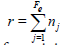is the number of failure units and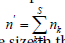is the number of surviving units, where n=r+n’ is the sample size in the test experiment.

The EM algorithm for estimating parameters is a general method for optimizing a log-likelihood function . Given a current estimate θ(h) we define the expectation of a log-likelihood function for grouped data that include exact times-to-failure and censoring as follows.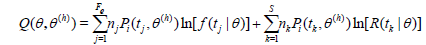(6)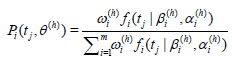is the posterior probability of a unit that belongs to the ith sub-population (i=1,2,….,m), which failed at time tj and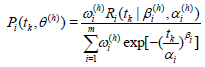is the conditional probability of a unit that belongs to subpopulation i given that it survived until time tk.

The EM algorithm is based on two main steps. The E step estimates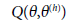and the M step selects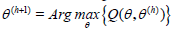by equating the first derivatives ofwith respect to each parameter with zero, thereby obtaining the following recurrence relations.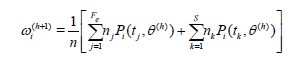(7)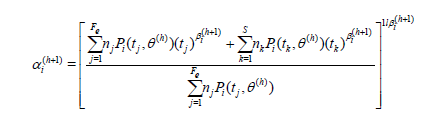(8)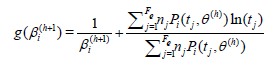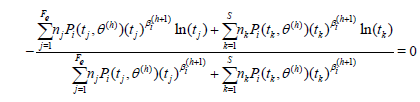(9)

These two steps are repeated alternately until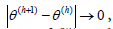which will be accurate if we select a good initial estimate of θ(h), and thus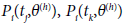will be obtained by solving Eq.(9) numerically using the Newton-Raphson method. After updating Eqs.(7),(8), and (9) many times, we can obtain MLE estimates of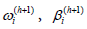and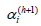for sub-population i.

Similarly, for complete and grouped ordered time-to-failure data, the last three equations can be written as follows.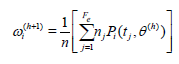(10)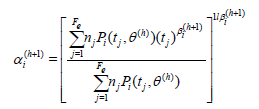(11)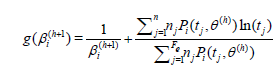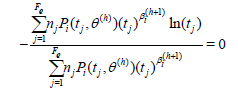(12)

As stated above, Eqs.(10), (11), and (12) can be solved numerically to estimate the parameters of the WMD model for complete lifetime data.

Algorithm 1: Proposed algorithm for estimating the wmd model parameters

The proposed algorithm for estimating the parameters of the WMD for modelling life data that include censored data can be summarized as follows:

Step 1: input given data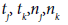Step 2: initialize parameters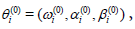define a convergence tolerance ε>0

Step 3: let h=0

Step 4: compute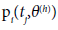and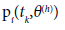Step 5: let h=h+1

Step 6: compute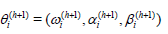Step 7: if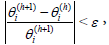stop,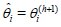where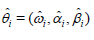Step 8: if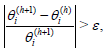goto step 4

Step 9: compute the log-likelihood function l

Step10: display the estimated parameters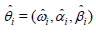Note: This algorithm was executed using MATLAB code. It also can be modified to estimate the parameters for a WMD model of complete failure data by making slight changes to some of the steps as follows:

Step 1: input given data tj, n

Step 4: compute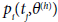NLMRR for estimating the WMD model parameters

In this section the optimization can be implemented using the Levenberg-Marquardt algorithm. The Levenberg-Marquardt algorithm is a non-linear iterative optimization method that can be used to minimize the sum of squares for the residuals due to error, SSR [18-20]. When regression analysis is applied to the WMD model to estimate its parameters, MATLAB program can be used for non-linear median rank regression, which is based on the modified Levenberg-Marquardt algorithm and median rank method. Estimates of the parameters in Eq.(4) are required to fit lifetime data with the WMD model. These parameters can be evaluated by using SSR, which can be defined as: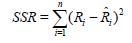(13)

where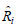denotes the approximated value of the reliability function which can be calculated using Eq.(4), and Ri is the actual value of the reliability function at ti, which can be determined by plotting a probability graph for the given lifetime data on WPP using various methods, such as the median rank method, Kaplan–Meier, or Benard’s median rank [6,10]. The required parameter estimates θ are the values that minimize SSR.

Eq. (13) can be written as: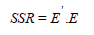(14)

where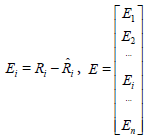and E’ is the transpose of E

Marquardt techniques depends on finding the gradient of SSR with respect to the set of parameters θ as follows: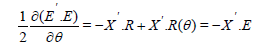(15)

where X is m×n matrix including the partial derivatives of R with respect to the parameters,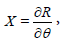and E is n×1 matrix including the error at each data point. The gradient method can be used to determine the best direction of moving in the θi space to obtain the smallest sum of squares for the residuals due to error as follows: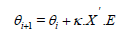(16)

Where κ is a control variable adjusts how far to move in the direction opposite to the gradient for updating the parameter values, but this method can not specify how far to move for finding the optimal solution. Levenberg-Marquardt technique treats this problem by using Gauss-Newton method. This method assumes that R(θ) can be expanded in θi space by using Taylor series about θ0 as follows: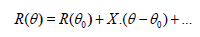(17)

By taking under consideration only the linear terms in the above equation and assuming that θ are the exact parameter values i.e there’s no error, therfore by the aids of Eq. (15) , one can deduce that: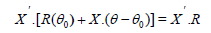(18)

Consequently, the updating formula of Gauss-Newton method can be written as: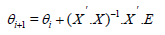(19)

The Levenberg-Marquardt algorithm combines these two methods through the following general formula: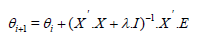(20)

where λ is the scaling parameter which balances the gradient-steepest-decent and Gauss-Newton methods. The optimal solution is obtained by adjusting λ and taking a good initial values for the parameters. The Levenberg-Marquardt algorithm is a stable, efficient and easily programmable.

#### Monte Carlo Simulation Study

In this section, analytical methods are compared for modelling the same system of components (units) using the Monte Carlo simulation technique. Monte Carlo simulation is based on the generally accepted belief that the probability distribution parameters obtained from simulations approximate their “true” values extremely well if the trials (replicates) are sufficiently long. Monte Carlo simulation method can be used to generate samples of different sizes from Weibull mixture models with given initial parameters [6,18]. But, before considering the Monte Carlo simulation technique, some concepts related to the estimator are defined and how one can choose a good estimator. An estimator is a mapping or a function from the data space to the parameter space, where the data space is the set of all possible values for a random sample with a certain size, and the parameter space is the set of all possible values of a parameter. The estimator is considerd as a sample statistic because it depends only on a given sample. It’s also considerd as a random variable for a random sample, and thus its value varies among samples according to its sampling distribution. Often, four major criteria are used to assess the performance of the estimator: bias, standard deviation, mean squared error, and efficiency.

Criterion for unbiasedness

sample statistic is an unbiased estimator if the mean or expected value of all possible values for the statistic equals the true or target value of the population parameter that the statistic attempts to estimate. If the mean or expected value of a statistic, which is produced by repeated random sampling from a given population, differs from the target that needs to be estimated, then bias is said to exist. Let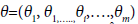be the parameter vector of an m-mixed Weibull distribution. If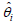is used to estimate the parameter θi, where θi∈θ, then the bias is defined as follows.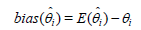(21)

The mean squared error (MSE) of the estimatoris given by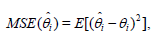(22)

which after some algebra can be written as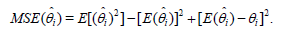(23)

Thus, the following relation can be deduced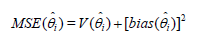(24)

Suppose that and are two estimators, for the same parameter θ. If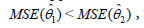thenis said to be more efficient than.

The comparison between the two analytical methods for estimating the parameters of WMD models, the maximum likelihood estimation (MLE) method using the expectation-maximization (EM) algorithm and the non-linear median rank regression (NLMRR) method with the Levenberg-Marquardt algorithm will be investigated in the following applications by using MATLAB program.

#### Applications

The Monte Carlo simulation method can be used to determine the best estimator for each parameter of the WMD among the proposed estimation methods. In this section, the use of the Monte Carlo simulation method is described to generate data samples of different sizes, which followed the two-component WMD. The aim of this study was to compare the performance of NLMRR using the Levenberg-Marquardt algorithm and MLE with the EM-algorithm. The simulation experiment was based on 1000 Monte Carlo trials (replicates) employing samples of sizes 10, 50, 100, and 500 failures, and 500 Monte Carlo trials with a sample size of 1000 and 5000 failures. In this experiment the WMD true parameters are choosen as ω1=0.3, β1=0.6, α1=39, ω2=0.7, β2=2.3, α2=234 . The two methods were compared using effective statistical measures, i.e., average parameter estimate, mean bias, standard deviation, and root mean squared error (RMSE). Tables 1-3 show the average parameter estimates obtained using the two methods for complete and censored samples, respectively. Tables 2 and 4 present the RMSE estimates obtained by the two methods for complete and censored samples, respectively. Tables 1 and 3 show clearly that for small or moderate complete and censored samples (50 or less), respectively, the bias estimates for the average parameter estimates based on the true values obtained using NLMRR method were very large and highly skewed compared with the bias estimates with the MLE method. In addition, Table 1 shows that for large complete samples (more than 100), the NLMRR method converged faster than MLE with the EM algorithm. By contrast, Table 3 shows that for large samples that included censored data, MLE with the EM algorithm converged faster than the NLMRR method. Table 2 shows that for complete large samples, the RMSE values estimated using MLE with the EM algorithm could be smaller or larger than those estimated using the NLMRR method. A method that obtains smaller RMSE values is more suitable for parameter estimation, and thus both methods were reasonable for obtaining a suitable goodness of fit in different cases. Table 4 shows that for large samples that included censored data, the RMSE values estimated using MLE with the EM algorithm were smaller than those estimated using the NLMRR method. Thus, in general, we can say that MLE using the EM algorithm yields low bias, variance, and RMSE values for small, moderate, and large sample sizes with censored data compared with the NLMRR method, and thus efficient parameter estimates were obtained for the WMD model in these cases. Using the Monte Carlo simulation method, one can modify the parameters of the specified life distribution and repeat simulations until an acceptable test plan is obtained. Figure 1 compares the CDFs for simulated complete failure data that followed the WMD obtained using the NLMRR method and MLE with the EM algorithm. In addition, Figure 2 shows the results obtained using failure data with heavily right censored data that followed the WMD. Each figure is divided into 4×2 subfigures, where those on the left show the results obtained using the NLMRR method and those on the right illustrate the results produced by MLE with the EM algorithm. In each subfigure, the solid black line denotes the true parameter line, the red dashed line is the average, and the blue lines represent the simulated lines based on several replicates. In Figures 1 and 2, the WMD models obtained using both methods for small, moderate, and large sample sizes with complete/censored data are compared. It was found that the WMD functions obtained using MLE with the EM algorithm based on complete failure data samples with small or moderate samples sizes and with large sample sizes that included censored data yielded the best fit. Thus, we conclude that the performance of MLE with the EM algorithm was much better for small or moderate complete failure data samples and large life data samples with a heavily censored data than the NLMRR method at estimating the WMD parameters.

Sample size Average estimates of parameter vector
NLMRR MLE through EM algorithm
10 (0.4337, 2.8238, 2.60E+18, 0.567, 7.6407, 8.00E+17) (0.3393, 3.1393, 38.2735, 0.6607, 4.9121, 247.97)
50 (0.3772, 0.7216, 446.2381, 0.6228, 3.403, 2.00E+17) (0.2483, 0.9175, 24.6031, 0.7517, 2.4119, 230.541)
100 (0.3493, 0.6805, 58.6739, 0.6507, 2.6795, 232.0602) (0.2380, 0.7647, 23.3483, 0.762, 2.2127, 229.064)
1000 (0.3043, 0.6182, 42.393, 0.6957, 2.3342, 232.2282) (0.2610, 0.6362, 29.4686, 0.739, 2.1826, 230.0765)
5000 (0.2960, 0.6119, 38.3756, 0.7040, 2.2984, 233.3096) (0.2943, 0.5997, 37.7418, 0.7057, 2.2791, 233.1497)

Table 1: Average parameter estimates: NLMRR versus MLE with the EM algorithm. WMD true parameters: ω1=0.3, β1=0.6, α1=39, ω2=0.7, β2=2.3, α2=234.

Sample size Root mean squared Error of parameter vector
NLMRR MLE through EM algorithm
100 (0.1421, O. 4119, 43. 2610, O. 1421, 0.9470, 19. 9987) (0.1090, O. 2911, 24. 5614, O. 1090, 0.4786, 19. 1756)
1000 (0.0608, 0.0633, 18. 5997, 0.0608, O. 2053, 6. 2323) (0.0646, 0.0753, 15. 5897, 0.0646, O. 2286, 7. 9292)
5000 (0.0360, 0.0329, 10.7732, O. 0360, O. 1001, 2.7935) (0.0180, O. 0179, 4. 4775, 0.0180, O. 0646, 2.5813)

Table 2: RMSE NLMRR versus MLE with the EM algorithm. WMD true parameters: ω1=0.3, β1=0.6, α1=39, ω2=0.7, β2=2.3, α2=234.

Sample size Average estimates of parameter vector
NLMRR MLE through EM algorithm
10 (0.2739, 11.5846, 1.31E+19, 0.7261, 12. 6615, 2.02E+17) (0.2887, 2.4142, 55. 8487, 0.7113, 15.3347, 154. 047)
50 (0.3556, 1. 5458, 2.00E+17, 0.6444, 5. 1881, 1. 05E+12) (0.3214, 0.9615, 66242.4005, 0.6786, 5. 1926, 206. 8489)
100 (0.3595, 0.903, 844341. 8693, 0.6405, 4. 0972, 1. 69E+06) (0.3322, 0.7445, 111. 3323, 0.6678, 3. 8833, 213. 2534)
1000 (0.3555, 0.6296, 91.4168, 0.6445, 2. 5049, 219. 5502) (0.3248, 0.607, 57.4823, 0.6752, 2. 3976, 228. 2388)
5000 (0.3360, 0.6018, 63.4317, 0.6640, 2. 3736, 228. 1733) (0.3143, 0.5951, 45. 5719, 0.6857, 2. 3292, 232. 8567)

Table 3: Average parameter estimates NLMRR versus MLE with EM algorithm for right censored life data. WMD true parameters: ω1=0.3, β1=0.6, α1=39, ω2=0.7, β2=2.3, α2=234.

Sample size Root Mean Squared Error of parameter vector
NLMRR MLE through EM algorithm
100 (0.2818, 1. 8905, 2.6114E+07, 0.2818, 6. 1644, 5.1928E+07) (0.2218, 0.3316, 277. 6568, 0.2218, 5. 2142, 51.0034)
1000 (0.2126, 0.0936, 132. 4833, 0.2126, 0.9970, 31.6453) (0.1137, 0.0654, 57. 2177, 0.1137, 0.5653, 16. 0803)
5000 (0.1239, 0.0431, 71. 3515, 0.1239, 0.3676, 15. 6561) (0.0466, 0.0254, 18. 3145, 0.0466, 0.1926, 6. 1047)

Table 4: RMSE values: NLMRR versus MLE with the EM algorithm for right censored life data. WMD true parameters: ω1=0.3, β1=0.6, α1=39, ω2=0.7, β2=2.3, α2=234.

Figure 1: Comparison of the CDFs obtained for simulated complete failure data that followed the WMD model with the following true parameters:Figure 2: Comparison of the CDFs obtained for simulated right censored failure data that followed the WMD model with the following true parameters:#### Conclusion

One of the aims of this paper is to apply algorithms that are stable, efficient and easily programmable for different Weibull models by using Matlab codes or any other specified programs that may be not found in libraries of some statistical packages software such as SPSS. These methods can be also extended and applied for different probability distribution models which are important task in reliability modeling. In this study, the CDFs obtained using MLE with the EM algorithm and the NLMRR method for simulated complete failure data or simulated failure data with heavily right censored data that followed the WMD model are compared. To perform this comparison, the Monte Carlo simulation method was used to generate data samples of different sizes, which followed the two-component WMD model. This simulation experiment was based on several Monte Carlo trials (replicates) for complete/censored failure samples with small, moderate, and large sizes. It was found that MLE with the EM algorithm achieved the lowest bias, variance, and RMSE values for small or moderate complete failure data samples. Moreover, for large samples that included censored data, MLE using the EM algorithm converged faster than the NLMRR method. Therefore, efficient parameter estimates for WMD models using MLE with the EM algorithm are obtained.

#### Acknowledgement

The author thanks the editor and the anonymous referees for their valuable comments and suggestions which have helped to greatly improve this paper. The author extends his appreciation to King Saud University, Deanship of Scientific Research, College of Science Research Center for supporting this project.

#### References

Select your language of interest to view the total content in your interested language

### Article Usage

• Total views: 10599
• [From(publication date):
June-2016 - Aug 25, 2019]
• Breakdown by view type
• HTML page views : 10383
• PDF downloads : 216

## Post your commentCan't read the image? click here to refresh
###### Peer Reviewed Journals

Make the best use of Scientific Research and information from our 700 + peer reviewed, Open Access Journals

International Conferences 2019-20

Meet Inspiring Speakers and Experts at our 3000+ Global Annual Meetings

Top